# Scientific Notation Scientific Notation Objective To identify standard

• Slides: 20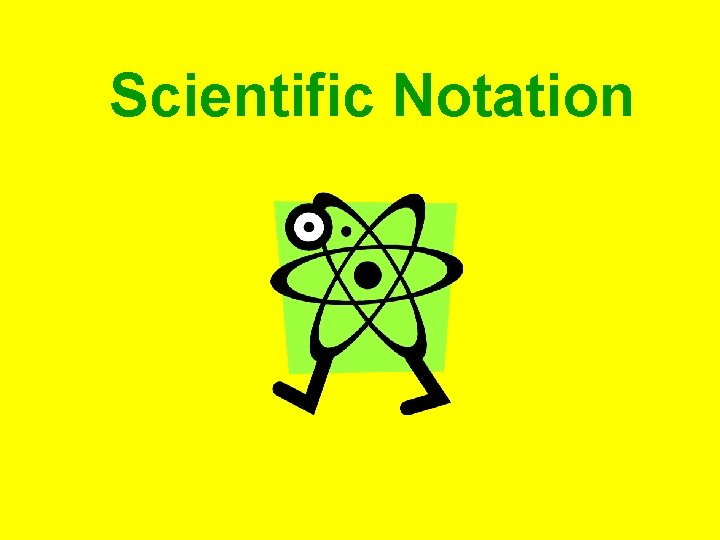Scientific NotationScientific Notation Objective: To identify standard form and scientific notation and be able to convert from one to the other with ease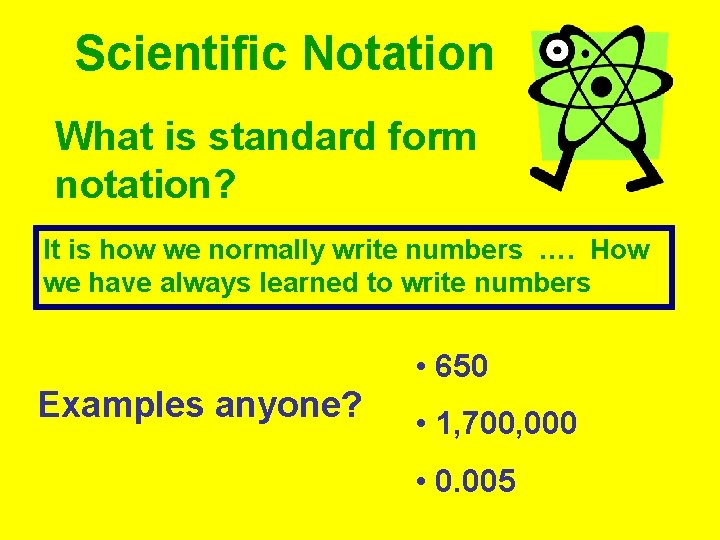Scientific Notation What is standard form notation? It is how we normally write numbers …. How we have always learned to write numbers • 650 Examples anyone? • 1, 700, 000 • 0. 005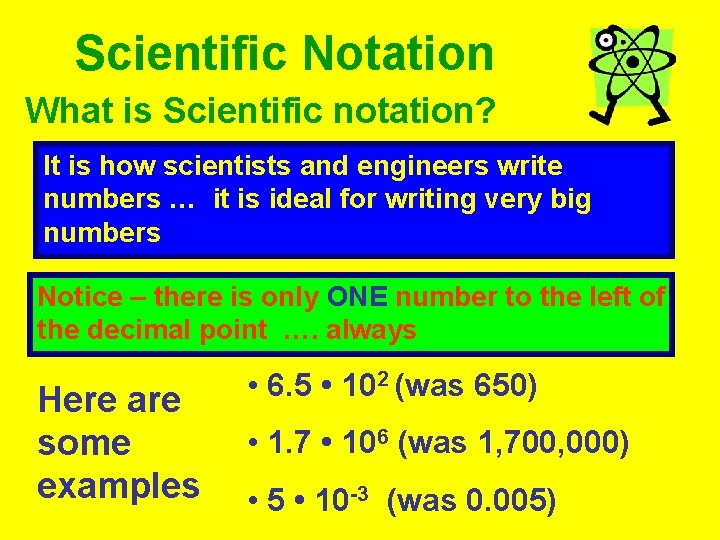Scientific Notation What is Scientific notation? It is how scientists and engineers write numbers … it is ideal for writing very big numbers Notice – there is only ONE number to the left of the decimal point …. always Here are some examples • 6. 5 • 102 (was 650) • 1. 7 • 106 (was 1, 700, 000) • 5 • 10 -3 (was 0. 005)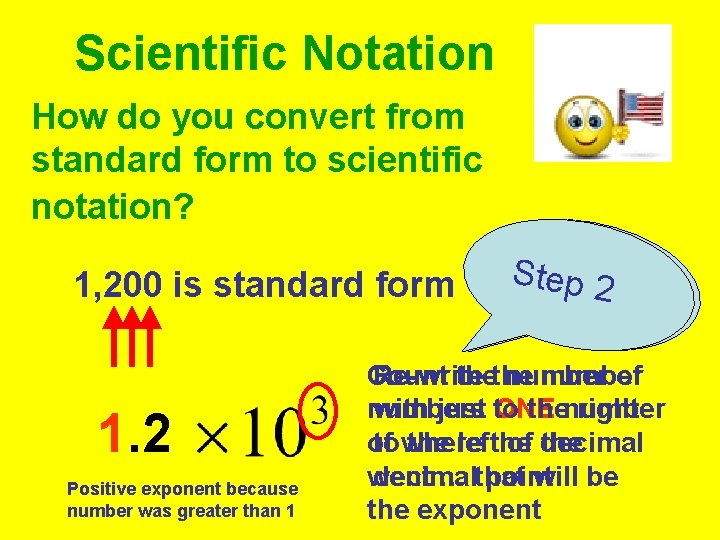Scientific Notation How do you convert from standard form to scientific notation? 1, 200 is standard form 1. 2 Positive exponent because number was greater than 1 SStteepp 21 Count Re-write thethe number of numbers with just to ONE thenumber right of to where the leftthe of decimal the went decimal … that point will be the exponent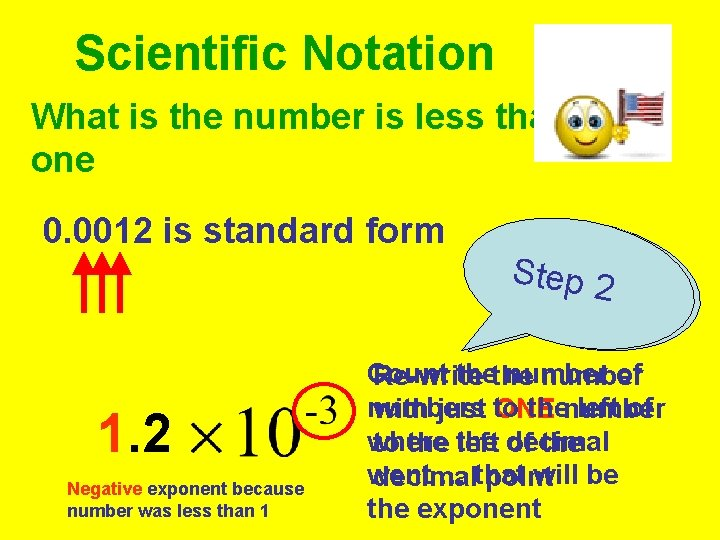Scientific Notation What is the number is less than one 0. 0012 is standard form 1. 2 Negative exponent because number was less than 1 SStteepp 21 Count thethe number of Re-write numbers thenumber left of with just to ONE where to the left decimal of the went … that will be decimal point the exponent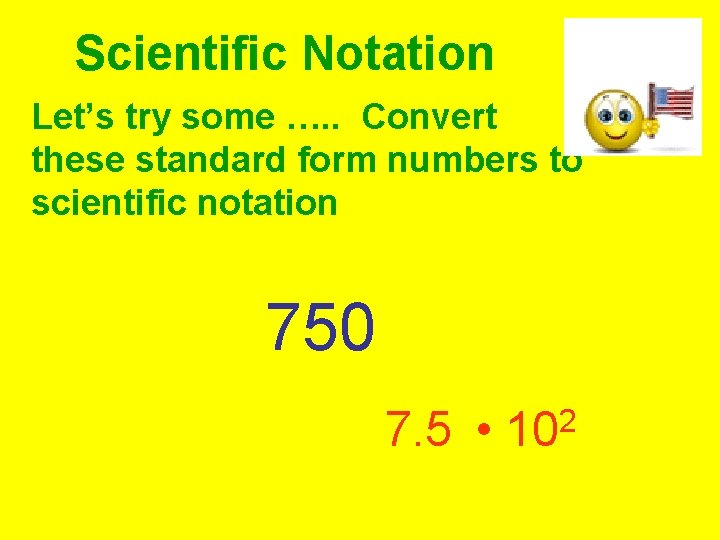Scientific Notation Let’s try some …. . Convert these standard form numbers to scientific notation 750 7. 5 • 2 10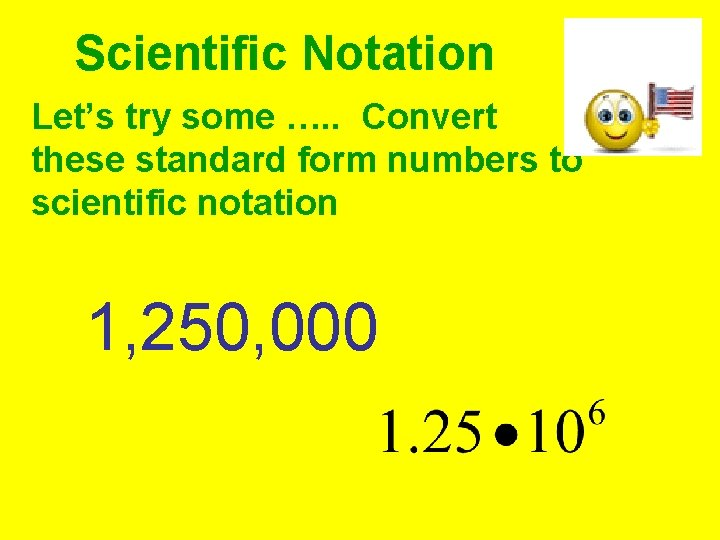Scientific Notation Let’s try some …. . Convert these standard form numbers to scientific notation 1, 250, 000Scientific Notation Let’s try some …. . Convert these standard form numbers to scientific notation 7500Scientific Notation Let’s try some …. . Convert these standard form numbers to scientific notation 0. 00015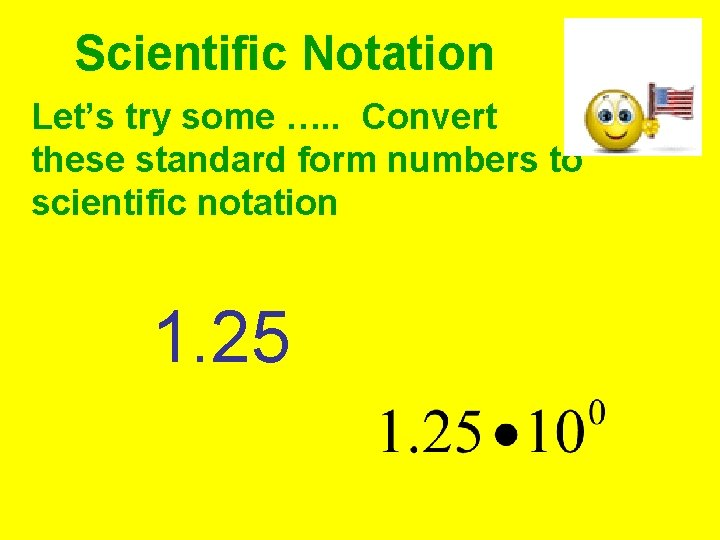Scientific Notation Let’s try some …. . Convert these standard form numbers to scientific notation 1. 25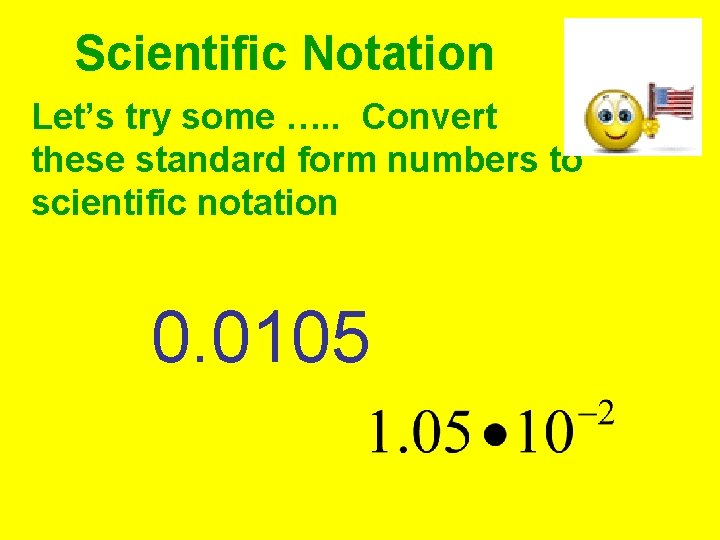Scientific Notation Let’s try some …. . Convert these standard form numbers to scientific notation 0. 0105Scientific Notation Let’s try some …. . Convert these standard form numbers to scientific notation 5, 025, 000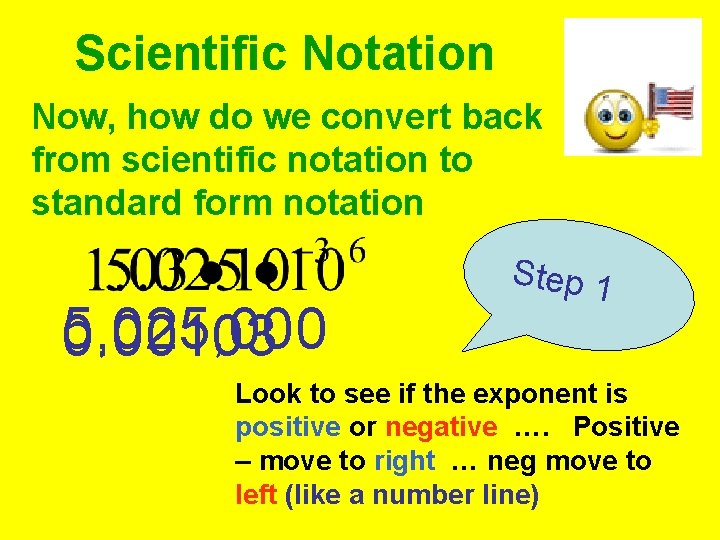Scientific Notation Now, how do we convert back from scientific notation to standard form notation 5, 025, 000 0. 00103 Step 1 Look to see if the exponent is positive or negative …. Positive – move to right … neg move to left (like a number line)Scientific Notation Now, how do we convert back from scientific notation to standard form notation 325, 000Scientific Notation Now, how do we convert back from scientific notation to standard form notation 1, 300Scientific Notation Now, how do we convert back from scientific notation to standard form notation 0. 00013Scientific Notation Now, how do we convert back from scientific notation to standard form notation 750. 3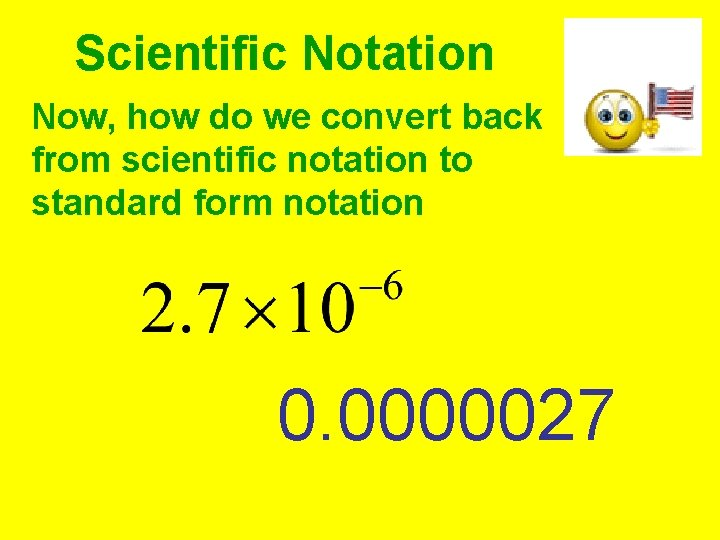Scientific Notation Now, how do we convert back from scientific notation to standard form notation 0. 0000027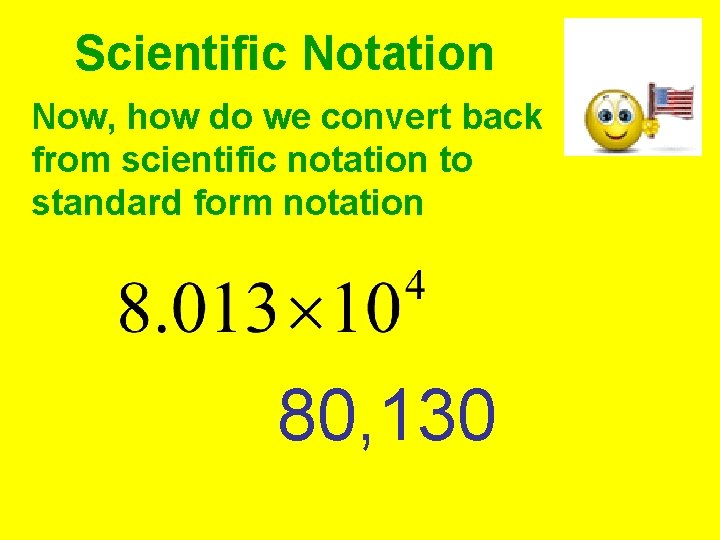Scientific Notation Now, how do we convert back from scientific notation to standard form notation 80, 130